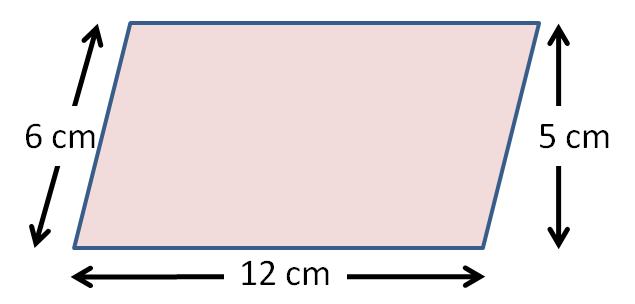• ## Accessibility

Open the accessibility toolbar to change fonts and contrast, access a dictionary, use a ruler and more

Parallelogram.  Another 2-dimensional shape that we easily recognise, but can't always remember its name.

It has opposite parallel sides, which may help with remembering the name.What examples can you think of that we see in everyday life?Probably not so obvious to think about.

Believe it or not, you can be two things at once!

For example, a square has two sets of parallel sides, so is also a parallelogram but we call it a square. The same goes for a rectangle.

Basically a parallelogram is a four-sided shape with their opposite sides equal in length (the same as a square or rectangle).Mathematicians can be awkward and make a four-sided look different just for fun, so that's how we arrive at parallelogram.

There are all sorts of reasons we may need to know the area of a parallelogram

Before we can do any of this there is one thing we need to know and that is how to find the area of a parallelogram.

First of all, we know that the sides of a parallelogram are not all the same length.

Two sides will have one length and the other two sides a different length.

As with a lot of shape work, there is a formula that you need to learn. So here goes:

Area of a parallelogram =base x height

You may recognise that it is the same as the formula for the area of a square and a rectangle.

Find the area of the parallelogram below.Area = base x height

Hang on!! We cannot work out the area of the parallelogram above as we only know the slanted height!Look out!  You need to work with the vertical height, not the slanted height.

12 x 5 = 60 cm²

As we are working with area units are always squared. e.g cm² m²

What a bargain - one formula for three shapes.

10 questions